# 1. 简介

LSTM模型作为一种经典的RNN网络结构，常用于NLP任务当中。在本篇工作中，我们进一步拓展了原始LSTM模型。注意到原始LSTM中输入x和之前状态h_prev是完全独立的，可能导致上下文信息的流失。我们提出一种形变LSTM，将输入x和之前状态h_prev进行交互，再输入进各个门里面运算。最后实验表明，改进过后的Mogrifier LSTM在各项任务均优于传统LSTM

# 2. 回顾传统LSTM

LSTM模型结构如下所示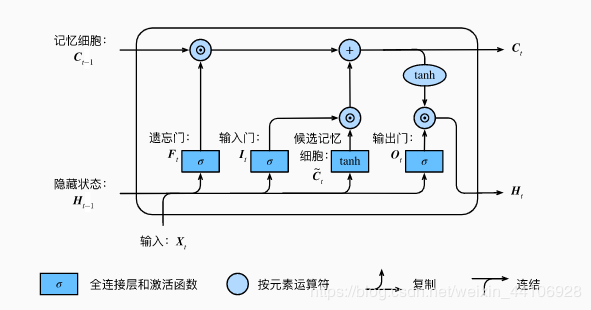• 遗忘门:

• 输入门:

• 候选记忆细胞:

• 输出门:

• 记忆细胞:

• 新一轮的隐藏状态:

• 遗忘门：主要控制是否遗忘上一层的记忆细胞状态， 输入分别是当前时间步序列数据，上一时间步的隐藏状态，进行矩阵相乘，经sigmoid激活后，获得一个值域在[0, 1]的输出F，再跟上一层记忆细胞进行对应元素相乘，输出F中越接近0，代表需要遗忘上层记忆细胞的元素。

• 候选记忆细胞：这里的区别在于将sigmoid函数换成tanh激活函数，因此输出的值域在[-1, 1]。

• 输入门：与遗忘门类似，也是经过sigmoid激活后，获得一个值域在[0, 1]的输出。它用于控制当前输入X经过候选记忆细胞如何流入当前时间步的记忆细胞。 如果输入门输出接近为0，而遗忘门接近为1，则当前记忆细胞一直保存过去状态

• 输出门：也是通过sigmoid激活，获得一个值域在[0,1]的输出。主要控制记忆细胞到下一时间步隐藏状态的信息流动

# 3. Mogrifier LSTM

Mogrifier LSTM引入以下两个公式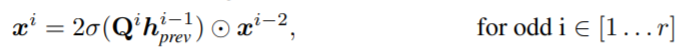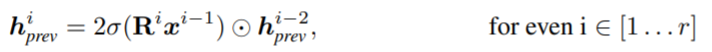，整个模型就退化成原始的LSTM

# 4. 实验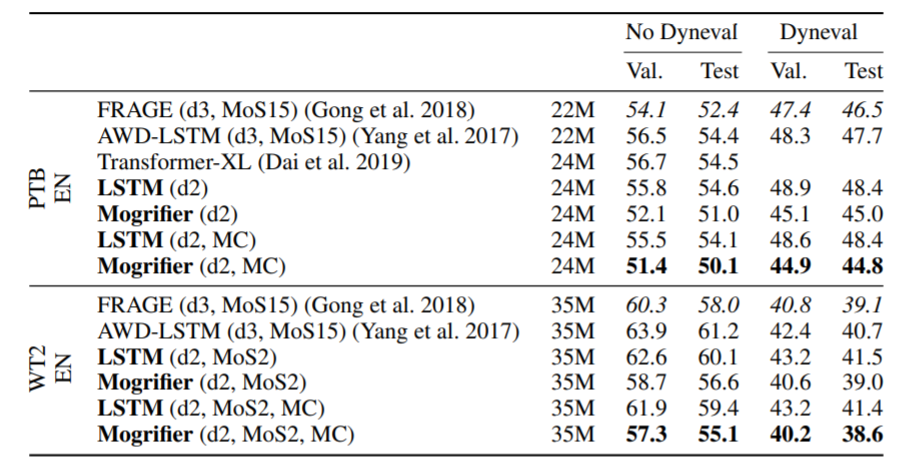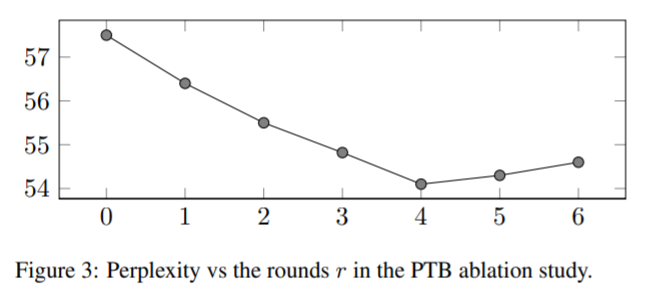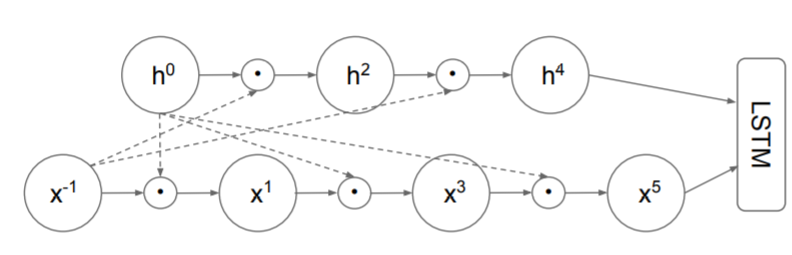# 5. 代码解析

class MogLSTM(nn.Module):
def __init__(self, input_sz, hidden_sz, mog_iteration):
super(MogLSTM, self).__init__()
self.input_size = input_sz
self.hidden_size = hidden_sz
self.mog_iterations = mog_iteration

# 这里hiddensz乘4，是将四个门的张量运算都合并到一个矩阵当中，后续再通过张量分块给每个门
self.Wih = Parameter(torch.Tensor(input_sz, hidden_sz*4))
self.Whh = Parameter(torch.Tensor(hidden_sz, hidden_sz*4))
self.bih = Parameter(torch.Tensor(hidden_sz*4))
self.bhh = Parameter(torch.Tensor(hidden_sz*4))

# Mogrifiers
self.Q = Parameter(torch.Tensor(hidden_sz, input_sz))
self.R = Parameter(torch.Tensor(input_sz, hidden_sz))

self.init_weights()

def init_weights(self):
"""
权重初始化，对于W,Q,R使用xavier
对于偏置b则使用0初始化
:return:
"""
for p in self.parameters():
if p.data.ndimension() >= 2:
nn.init.xavier_uniform_(p.data)
else:
nn.init.zeros_(p.data)

def mogrify(self, xt, ht):
"""
计算mogrify
:param xt:
:param ht:
:return:
"""
for i in range(1, self.mog_iterations+1):
if(i % 2 == 0):
ht = (2*torch.sigmoid(xt @ self.R)*ht)
else:
xt = (2*torch.sigmoid(ht @ self.Q)*xt)
return xt, ht

def forward(self, x:torch.Tensor, init_states:Optional[Tuple[torch.Tensor, torch.Tensor]]=None) -> \
Tuple[torch.Tensor, Tuple[torch.Tensor, torch.Tensor]]:
batch_sz, seq_sz, _ = x.size()
hidden_seq = []
if init_states is None:
ht = torch.zeros((batch_sz, self.hidden_size)).to(x.device)
Ct = torch.zeros((batch_sz, self.hidden_size)).to(x.device)
else:
ht, Ct = init_states

for t in range(seq_sz):
xt = x[:, t, :]
xt, ht = self.mogrify(xt, ht)
gates = (xt @ self.Wih + self.bih) + (ht @ self.Whh + self.bhh)
ingate, forgetgate, cellgate, outgate = gates.chunk(4, 1) # chunk方法将tensor分块

# LSTM
ft = torch.sigmoid(forgetgate)
it = torch.sigmoid(ingate)
Ct_candidate = torch.tanh(cellgate)
ot = torch.sigmoid(outgate)
# outputs
Ct = (ft*Ct) + (it*Ct_candidate)
ht = ot * torch.tanh(Ct)
hidden_seq.append(ht.unsqueeze(Dim.batch)) # unsqueeze是给指定位置加上维数为1的维度
hidden_seq = torch.cat(hidden_seq, dim=Dim.batch)
hidden_seq = hidden_seq.transpose(Dim.batch, Dim.seq).contiguous()
return hidden_seq, (ht, Ct)

• 首先输入分别表示 输入维度，隐层维度，Mogrify的计算次数（也就是前面提到的超参数i）

• 然后分别初始化 权重Wih, Whh,Bih,Bhh。注意这里要乘4，这是因为LSTM里面有4个门，它将其合并为一个矩阵运算，最后再分配给4个门，提高速度

• 同样也是随机初始化用于Mogrify计算的两个矩阵Q ，R

• init_weights是进行参数初始化

• 方法mogrify里面，就是mogrify计算的部分了，根据计算次数设定一个for循环，根据奇偶性，分别对X和H进行交互计算，并返回

• 在forward前向计算中，先对隐层和输入X做初始化。然后进行矩阵运算，通过chunks方法将张量分成4部分，分别给四个门，再根据我们的前面的公式，分别进行sigmoid和tanh计算。然后更新细胞状态和隐藏状态。将隐藏状态连结成序列，最终返回隐藏状态序列，隐藏状态和细胞状态

# 6. 总结11-2807-261万+
10-28395
01-161万+
10-308539
03-051675
©️2020 CSDN 皮肤主题: Age of Ai 设计师:meimeiellie